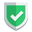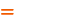我们相信：世界是美好的，你是我也是。平行空间的世界里面，不同版本的生活也在继续...

`python`代码里面有个`dir()`函数，那么这里面的`dir`函数和大家已经熟悉的`dir`命令和`ls`命令，有什么关系呢？本文的主要内容就是看看`python`里面的`dir`函数，究竟是用来做什么用的呢？是用于列出某个目录下面的所有文件么？请参考本文中的内容。

## `dir()`列出当前环境变量

``````a = (1,)
b = [1, ]
print(__file__)
print(__name__)
print(dir())``````

``````c:/Users/sunan/Desktop/py/test.py
__main__
['__annotations__', '__builtins__', '__cached__', '__doc__', '__file__', '__loader__', '__name__', '__package__', '__spec__', 'a', 'b']``````

## `dir(arg)`列出当前变量的可用操作

``````a = (1,)
b = [1, ]
print(dir(a))``````

``['__add__', '__class__', '__contains__', '__delattr__', '__dir__', '__doc__', '__eq__', '__format__', '__ge__', '__getattribute__', '__getitem__', '__getnewargs__', '__gt__', '__hash__', '__init__', '__init_subclass__', '__iter__', '__le__', '__len__', '__lt__', '__mul__', '__ne__', '__new__', '__reduce__', '__reduce_ex__', '__repr__', '__rmul__', '__setattr__', '__sizeof__', '__str__', '__subclasshook__', 'count', 'index']``

## `listdir(path)`列出当前目录下文件

`python`中，列出当前目录下的文件的话，使用的是`OS`模块下的`listdir`函数。测试代码如下：

``````import os
cwd = os.path.dirname(__file__)
list = os.listdir(cwd)
print(list)``````

``['test.py', 'zip.zip', 'sunan.rtf', 'xls.xls', '新建文件夹']``

## 综述如果本文对您有帮助，或者节约了您的时间，欢迎打赏瓶饮料，建立下友谊关系。本博客不欢迎：各种镜像采集行为。请尊重原创文章内容，转载请保留作者链接。【福利】 腾讯云最新爆款活动！1核2G云服务器首年50元！【源码】本文代码片段及相关软件，请点此获取更多信息【绝密】秘籍文章入口，仅传授于有缘之人python